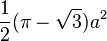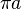# Reuleaux triangle facts for kids

Kids Encyclopedia Facts

A Reuleaux triangle is a shape made when three circles cross. It looks like a triangle with round sides and pointed corners.

To make one, first draw two dots. Then put a compass on the two dots and draw a circle. Do the same thing again, this time with the other dot as the middle of the circle. Choose either the top or bottom points of the connecting circles as a new center, using the two drawn dots as the edge, and draw the third circle.

If a is how wide the Reuleaux triangle is, the area is$\frac{1}{2}(\pi - \sqrt3)a^2$. The perimeter is$\pi a$.

A Reuleaux polygon is a shape like the Reuleaux triangle with an odd number of sides.

A Reuleaux tetrahedron is made when four spheres cross. It looks like a Reuleaux triangle as a pyramid with three sides at the bottom.

## Images for kidsReuleaux triangle Facts for Kids. Kiddle Encyclopedia.